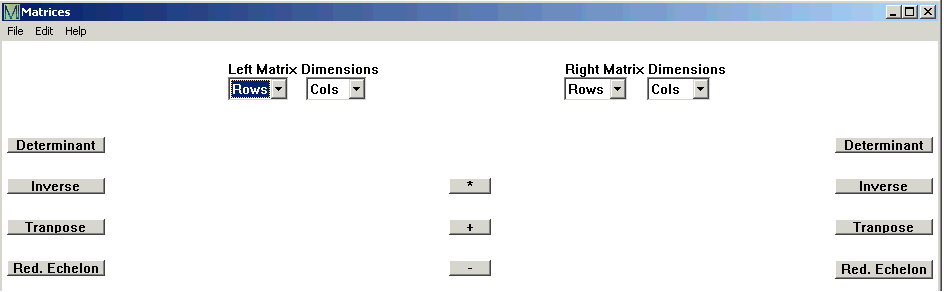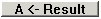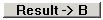# Click Linear Algebra and then Matrices. This window opens.A matrix is a rectangle of numbers; you begin by telling CalGraph how big the matrix is.

Enter the number of rows (the horizontals) in the Rows box under Left Matrix Dimensions.

Enter the number of Columns (the verticals) in the Cols box. You can have up to 10 rows, up to 10 columns.

Boxes open for the matrix. You type numbers in each box.If the matrix has a lot of the same number, it may be faster to fill every box with that number and then change the values you need to. On one side is a button called Fill. Enter the repeated number in the box under the Fill button and click Fill.

If you have a second matrix to work with, enter its number of rows under  Right Matrix Dimensions, and then its number of columns in the Cols box. Again you can use Fill, or you can type the values in each box.

Now you can calculate:

Determinant

Inverse

Transpose

Reduced Row Echelon Form

Multiply by a number

Multiply by a matrix

Subtract two matrices

You may have several calculations to do.

Clickto put an answer into the matrix on the left,

orto put the answer in the matrix on the right.

You can print your results by clicking File and then Print at the top of the window.

Or you can copy and paste them to another program using Edit Copy.